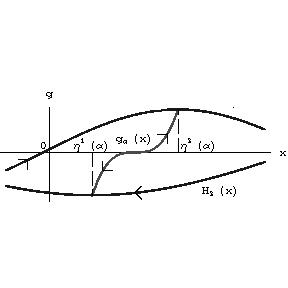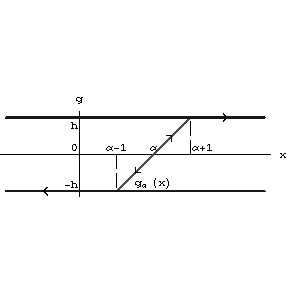# Hysteron

Only a very simple modification of the non-linearity "hysteron" is described below. See [a1] for the general definition and an identification theorem, that is, qualitative conditions under which a "black box" is a hysteron. Consider in the $( x,g )$- plane the graphs of two continuous functions $H _ {1} ( x )$, $H _ {2} ( x )$ satisfying the inequality $H _ {1} ( x ) < H _ {2} ( x )$, $x \in \mathbf R$. Suppose that the set $\Omega = \{ {\{ x,g \} } : {x \in \mathbf R, H _ {1} ( x ) \leq g \leq H _ {2} ( x ) } \}$ is partitioned into the disjoint union of the continuous family of graphs of continuous functions $g _ \alpha ( x )$, where $\alpha$ is a parameter. Each function $g _ \alpha ( x )$ is defined on its interval $[ \eta _ \alpha ^ {1} , \eta _ \alpha ^ {2} ]$, $\eta _ \alpha ^ {1} < \eta _ \alpha ^ {2}$, and $g _ \alpha ( \eta _ \alpha ^ {1} ) = H _ {1} ( \eta _ \alpha ^ {1} )$, $g _ \alpha ( \eta _ \alpha ^ {2} ) = H _ {2} ( \eta _ \alpha ^ {2} )$, that is, the end-points of the graphs of the functions $g _ \alpha ( x )$ belong to the union of the graphs of $H _ {1} ( x )$ and $H _ {2} ( x )$( see Fig.a2.).Figure: h110430aFigure: h110430b

Hysteron: Prandtl non-linearity

A hysteron is a transducer with internal states $\xi$ from the segment $[ 0,1 ]$ and with the following input–output operators. The variable output ${\mathcal H} ( \xi _ {0} ) x ( t ) \equiv {\mathcal H} ( \xi _ {0} ,t _ {0} ) x ( t )$( $t \geq t _ {0}$) is defined by the formula

$${\mathcal H} ( \xi _ {0} ) x ( t ) = \left \{ \begin{array}{l} {g _ \alpha ( x ( t ) ) , \ \eta _ \alpha ^ {1} \leq x ( t ) \leq \eta _ \alpha ^ {2} , } \\ {H _ {1} ( x ( t ) ) , \ x ( t ) \leq \eta _ \alpha ^ {1} , } \\ {H _ {2} ( x ( t ) ) , \ \eta _ \alpha ^ {2} \leq x ( t ) , } \end{array} \right .$$

for monotone inputs $x ( t )$, $t \geq t _ {0}$. The value of $\alpha$ is determined by the initial state $\xi$ to satisfy $g _ \alpha ( x ( t _ {0} ) ) = \xi _ {0} H _ {1} ( x ( t _ {0} ) ) + ( 1 - \xi _ {0} ) H _ {2} ( x ( t _ {0} ) )$ and the corresponding variable internal state is defined by

$$\Xi ( \xi _ {0} ) x ( t ) = { \frac{ {\mathcal H} ( \xi _ {0} ) x ( t ) - H _ {1} ( x ( t ) ) }{H _ {2} ( x ( t ) ) - H _ {1} ( x ( t ) ) } } .$$

For piecewise-monotone continuous inputs the output is constructed by the semi-group identity. The input–output operators can then be extended to the totality of all continuous inputs by continuity (see [a1]). The operators ${\mathcal H} ( \xi _ {0} ) x ( t )$, $\Xi ( \xi _ {0} ) x ( t )$ are defined for each continuous input and each initial state. They are continuous as operators in the space of continuous functions with the uniform metric (cf. also Metric).

A hysteron is called a Prandtl non-linearity if $H _ {1} ( x ) \equiv - h$, $H _ {2} ( x ) \equiv h$; $g _ \alpha = kx - \alpha$, $\alpha - h \leq x \leq \alpha + h$, $\alpha \in \mathbf R$. This non-linearity describes the Prandtl model of ideal plasticity with Young modulus $k$ and elastic limits $h$. The parallel connections of a finite numbers of such elements describe the Besseling model of elasto-plasticity and the continual counterpart describe the Ishlinskii model.